# Integration by substitution

Jump to: navigation, search
This article is about an integration rule, i.e., a rule for integrating a function expressed in terms of other related functions.
View other integration rules

## Definition

Integration by substitution is a general technique for finding antiderivatives of expressions that involve products and composites that works by trying to reverse-engineer the chain rule for differentiation.

### Indefinite integral version

Suppose we are trying to integrate an expression of the form: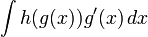$\int h(g(x))g'(x) \, dx$

Integration by substitution works by putting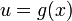$u = g(x)$ and solving the integration: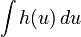$\int h(u) \, du$

If$f$ is an antiderivative for$h$, so that$f'(u) = h(u)$, then an antiderivative for the original expression (after we back-substitute$u = g(x)$) is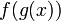$f(g(x))$. The indefinite integral is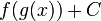$f(g(x)) + C$.

Because the variable used for substitution is conventionally taken as$u$ (as in the setup above), integration by substitution is often called integration by$u$-substitution.

### Justification in terms of chain rule

Note that the reason integration by substitution works is that it reverses the chain rule for differentiation: if we know that$f' = h$, then:$\frac{d}{dx}[f(g(x))] = f'(g(x))g'(x) = h(g(x))g'(x)$

So in this sense, integration by substitution is not giving us any new information beyond what we already knew from the chain rule. The main advantage is that, by doing the substitution, we are able to break down our problem into separate steps and thereby make each step individually simpler and more tractable.

### Definite integral version

There are two ways that we can use integration by substitution to carry out definite integrals. One is that we simply use it to complete the indefinite integration, and then plug in and evaluate between limits. However, there is another version that is specifically adapted to definite integration. This says that when making the substitution, also change the limits of integration according to the substituting function. If we do this, we do not need to substitute back in terms of the original variable at the end. Instead, we simply compute the new definite integral. In symbols:$\int_a^b h(g(x))g'(x) \, dx \stackrel{u = g(x)}{=} \int_{g(a)}^{g(b)} h(u) \, du$

The definite integral version thus involves changing limits as we go along. This has a number of advantages, one of which is that it may be possible to compute the definite integral using symmetries and other methods specific to definite integrals, even though the antiderivative cannot be computed within the functions that we're familiar with.

## Examples

### Example of integration by substitution: indefinite integral version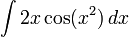$\int 2x \cos (x^2) \, dx$

Here,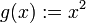$g(x) := x^2$,$h = \cos$, and we see that we are in the setup originally mentioned. Thus, we do the substitution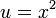$u = x^2$. The part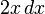$2x \, dx$ gets replaced by$du$, and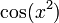$\cos(x^2)$ becomes$\cos u$, so we get:$\int \cos u \, du$

This simplifies to: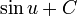$\sin u + C$

Substituting back, we get:$\sin(x^2) + C$

### Example of integration by substitution: definite integral version where antiderivative is not possible to compute

Consider the following integration problem:$\int_0^\pi e^{\cos^2x} \cos x \, dx$

We rewrite$\cos^2x = 1 - \sin^2x$ and put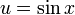$u = \sin x$ to get:$\int_{\sin 0}^{\sin \pi} e^{1 - u^2} \, du$

This becomes: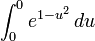$\int_0^0 e^{1 - u^2} \, du$

The antiderivative of$e^{1 - u^2}$ is not in the collection of elementarily expressible functions. However, we don't actually need to compute the antiderivative because we see that the upper and lower limits of integration are equal to each other, forcing the definite integral to be 0. Thus, the answer is:$0$